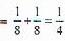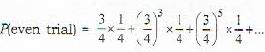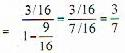Courses

# Test: Probability - 8

## 20 Questions MCQ Test Topic-wise Tests & Solved Examples for IIT JAM Mathematics | Test: Probability - 8

Description
This mock test of Test: Probability - 8 for Mathematics helps you for every Mathematics entrance exam. This contains 20 Multiple Choice Questions for Mathematics Test: Probability - 8 (mcq) to study with solutions a complete question bank. The solved questions answers in this Test: Probability - 8 quiz give you a good mix of easy questions and tough questions. Mathematics students definitely take this Test: Probability - 8 exercise for a better result in the exam. You can find other Test: Probability - 8 extra questions, long questions & short questions for Mathematics on EduRev as well by searching above.
QUESTION: 1

### Let A, B and C be independent and mutually exclusive events with probability of occurrences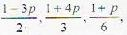respectively, then p lies in

Solution:

As probability of any event is greater than or equal to zero and sum of mutually exclusive events in less than or equal to one, so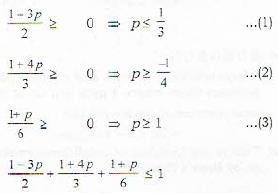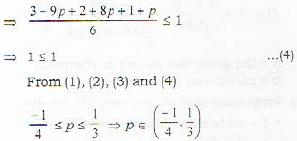QUESTION: 2

### A communication system consists of n components components. Each of these functions independently with probability p. The system function correctly if and only if at least half of its components functions. For what range of p, the probability that a five-components system functions correctly is higher than the probability that a three-components system functions correctly?

Solution:

Five component system functions correctly if at least 3 components function and 3 component system component function correctly if at least two components function.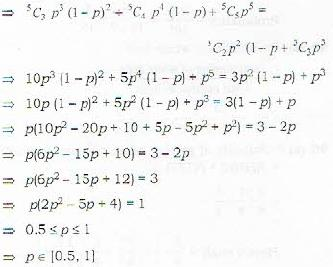QUESTION: 3

### The probability of getting a defective floppy in three boxes A, 13 and C arc 1/3, 1/6 and 3/4, respectively. A box is selected randomly and a floppy is drawn from it. The probability that the floppy is defective and is drawn from box A is

Solution:

It is conditional probability’s case where detective floppy can come from any box. It will come from box A with probability P (A | defective)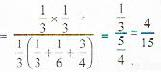QUESTION: 4

Let A and B be two events with P(A) = 1 /2, P(B) = 1 /3 and P(A ∩ B) = 1/4, What is P(A|B)?

Solution: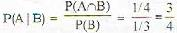QUESTION: 5

Let A and B be two events with P(A) =1/2, P(B) = 1/3 and P (A ∩ B) = 1/4, What is P (A ∪ B)?

Solution: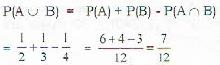QUESTION: 6

If the probability of a bad reaction from a certain injection is 0.001 then what is the chance that out of 2000 individuals more than two will get a bad injection?

Solution: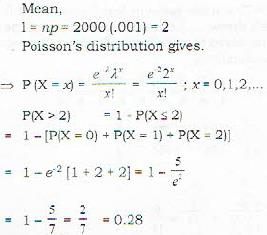QUESTION: 7

Let X, Y, Z be independent Poisson variables, such that E(X) = E(Y) and E[Z) = 2E(X). If P[X- 5, y = 4) is equal to P[Z = 8), then E(X) is

Solution: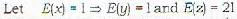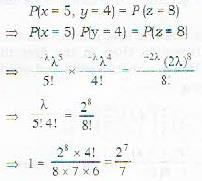QUESTION: 8

Two letters are chosen one after another without replacement from the English alphabet. What is the probability that the second letter chosen is a vowel?

Solution: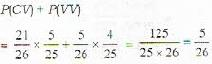Note : There are 21 cosonants and 5 vowels in 26 letters of alphabet.

QUESTION: 9

A cow is tied with a pole by a 100 meter long rope. What is the probability that at some point of time the cow is at least 60 meters away from the pole?

Solution: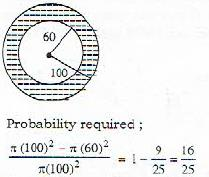QUESTION: 10

If two dice, are tossed the probability of getting the sum at least 5 is

Solution:

Sum of the number so n the face so f two dice, is 2, 3 and 4 in 1 , 2 and 3 cases respectively, so remaining 36-(1+2+3) - 30 cases are in our favour.
So, probability =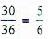QUESTION: 11

A and B play a game of dice. A throws che die first. The person who first gets a 6 is the winner. What is the probability that A wins?

Solution:

A will win the game in first throw, third throw, fifth throw , .....i.e. in odd throw provided the even numbered throw is lost by B. Hence the required probability is,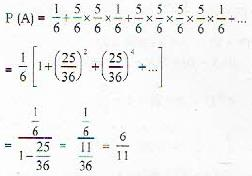QUESTION: 12

Two teams A and B play a series of four matches. If the probability that team A wins a match is 2/3,  then the probability that team A wins three matches, loses one and the third win occurs in the fourth match is

Solution:

In three wins and one loss if third win occurs in the fourth match, then in the firs t three match there was exactly two wins and one loss so probability is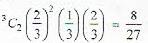QUESTION: 13

A fair coin is tossed twice. Let A be the event that at least one tail appears and B be the event that both head and tail appear. Then P[A/B], the probability of A given B, is

Solution: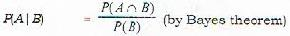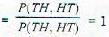QUESTION: 14

Let A and B be events with P(A) = 2/3, = P(B) = 1/2 and P(A∩B) = 1/3.  Match Lists 1 and II and select the correct answer: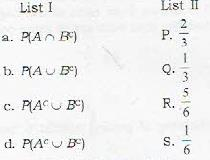Solution: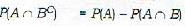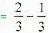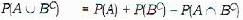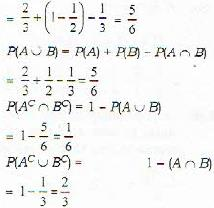QUESTION: 15

Total number of ways in which four boys and three girls can sit in a row so that boys and girls have alternate seats is

Solution:

B G B G B G B
4 boys will sit in 4! ways and create 3 places in between them where 3 girls will sit in 3! ways total number of ways = (4!) (3!)
= 24 x 6 = 144 Ans

QUESTION: 16

A player is going to play a match either in the morning or in the afternoon or in the evening all possibilities being equally likely. The probability that he wins the match is 0.6, 0.1 and 0.8 according as if the match is played in the morning, afternoon or in the evening respectively. Given that he has won the match, the probability that the match was played in the afternoon is

Solution:

This is the problem of conditional probability, so by Bave’s theorem.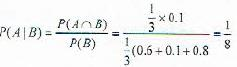A = the game was played in afternoonB B = player won the game.

QUESTION: 17

The probabilities that a husband and wife will be alive 20 years from now are given by 0.8 and 0.9 respectively. What is the probability that in 20 years at least one, will be alive?

Solution:

Probability that at least one will be alive
= 1 - probability that no one will be alive
= 1-(0.2)(0.1)
= 1 - 0.02 = 0.98

QUESTION: 18

A shelf has 6 mathematics books and 4 physics books. The probability that 3 particular mathematics books will be together is?

Solution:

The three particular books are treated as a unit which are arranged among the m selves after total 4 + 3 + 1 = 8 units have been arranged.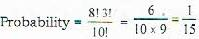QUESTION: 19

A marble is drawn at random from a box containing 10 red, 30 white, 20 blue and 15 orange marbles. What is the probability of the ball is white?

Solution:

Probability of wiiite ball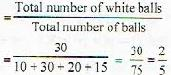QUESTION: 20

Three persons play a game by tossing a fair coin each independently. The game ends in a trial if all of them get the same outcome in that trial, otherwise they continue to the next trial. What is the probability that the game ends in an even number of trials?

Solution:

Probability of getting same outcomes in a trial = P(HHH) + P[TTT)